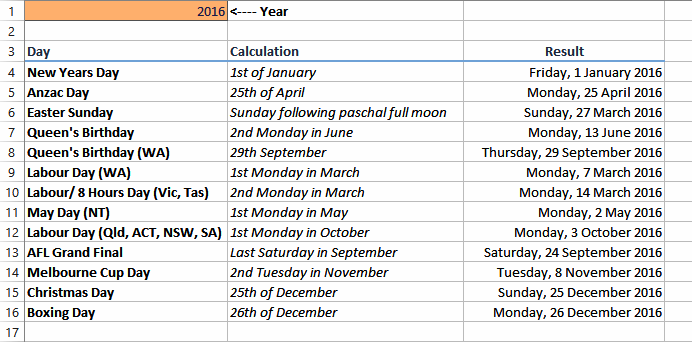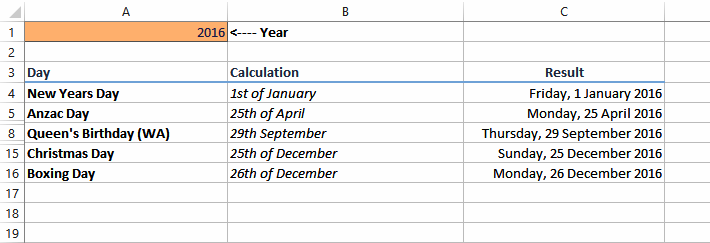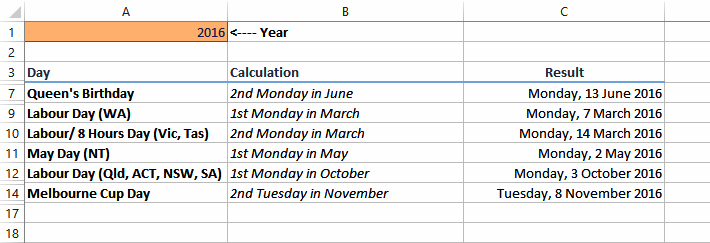Close

Note: This is the first of a two part series. You can view part 2 here.

No, we're not getting fresh! A common problem for many Excel users though is finding a date - not the romantic type of date but dates such as public holidays or the dates of recurring events such as monthly meetings and the like. So in the next few tech tips we'll show you how to calculate specific future dates.

Here's a sample of dates we'll show you how to calculate. They range from quite simple to extremely complex.### Recurring future dates

Recurring future dates are ones that occur based on a recurring and predictable pattern. There are several variants of these.

#### Fixed Day Dates

The simplest are those that are based upon a specific and easily identifiable day. Take Christmas Day or New Year's Day or even your birthday for example. We know when they occur in any particular year. So the simplest way to calculate this would be you use the DATE() function with its three paramaters: year, month, and day.Here we have standard holidays in Australia where the day is always the same for any given year. The formula in cell C4 to calculate New Years Day is:

```=DATE(A1, 1, 1)
```

The cell is formatted to include the day of the week.

The other days are calculated and formatted similarly:

```=DATE(A1, 4, 25) for Anzac Day
=DATE(A1, 9, 29) for Queen's Birthday (WA)
=DATE(A1, 12, 25) for Christmas Day
=DATE(A1, 12, 26) for Boxing Day
```

#### The First, Second, or Third Occurrence In A Month

These types of dates commonly include ones based on the first Monday, or the second Tuesday of a particular month in a given year.This type of calculation is more complex. Let's take the Queen's Birthday example above which is held on the second Monday of June. To calculate this for any given year we first begin by taking the date for June 1st, then adding the number of days until the second Monday in that month for that year.

In Excel the days of the week are given an index number starting from Sunday (1) through to the following Saturday (7). In this scheme Monday is day number 2 in the week. You can find the index number for any given date using the WEEKDAY() function which requires the serial number of the date.

So the logic for our Queen's Birthday calculation is to find the date for the first of the June then add the number of days to the second Monday to that date. The first part is achieved by using the DATE() function again.

Finding the number of days to the second Monday requires a bit more work (understatement!). We begin by finding the number of days to the first Monday as follows:

1. If the weekday index number of June 1 is less than the index number for Monday, we take the index number of June 1 away from 7 and add 2 (the index number for Monday)
2. If the weekday index number of June 1 is greater than the index number for Monday, we take the index number for June 1 away from 2 (the index number for Monday)

To express this in pseudo-Excel function language we'd use an IF() function as follows:

```IF(2 < WEEKDAY(June 1, 2016), 7 - WEEKDAY(June 1, 2016) + 2, 2 - WEEKDAY(June 1, 2016))
```

June 1, 2016, falls on a Wednesday which gives us an index number of 4. Placing the index number into this formula for 2016 gives us:

```IF(2 < 4, 7 - 4 + 2, 2 - 4)
```

which will return 5 (because with 2 being less than 4 the result of 7 - 4 + 2 equals 5).

So we know that the first Monday in June 1, 2016, occurs 5 days after the start of the month. To determine the second Monday we simply add another 7 days to this result. So the second Monday in June 2016 occurs 12 days after the start of the month.

In the above example we've used the following formula (assuming the year is in cell A1):

```=DATE(A1, 6, 1) + IF(2 < WEEKDAY(DATE(A1, 6, 1)), 7 - WEEKDAY(DATE(A1, 6, 1)) + 2, 2 - WEEKDAY(DATE(A1, 6, 1))) + 7
```

The formulas for the other dates follow a similar logic but are tweaked according to when the days fall:

#### Labour Day (WA) - 1st Monday in March

```=DATE(A1, 3, 1) + IF(2 < WEEKDAY(DATE(A1, 3, 1)), 7 - WEEKDAY(DATE(A1, 3, 1)) + 2, 2 - WEEKDAY(DATE(A1, 3, 1)))
```

#### Labour/ 8 Hours Day (Vic, Tas) - 2nd Monday in March

```=DATE(A1, 3, 1) + IF(2 < WEEKDAY(DATE(A1, 3, 1)), 7 - WEEKDAY(DATE(A1, 3, 1)) + 2, 2 - WEEKDAY(DATE(A1, 3, 1))) + 7
```

#### May Day (NT) - 1st Monday in May

```=DATE(A1, 5, 1) + IF(2 < WEEKDAY(DATE(A1, 5, 1)), 7 - WEEKDAY(DATE(A1, 5, 1)) + 2, 2 - WEEKDAY(DATE(A1, 5, 1)))
```

#### Labour Day (Qld, ACT, NSW, SA) - 1st Monday in October

```=DATE(A1, 10, 1) + IF(2 < WEEKDAY(DATE(A1, 10, 1)), 7 - WEEKDAY(DATE(A1, 10, 1)) + 2, 2 - WEEKDAY(DATE(A1, 10, 1)))
```

#### Melbourne Cup Day - 2nd Tuesday in November

```=DATE(A1, 11, 1) + IF(3 < WEEKDAY(DATE(A1, 11, 1)), 7 - WEEKDAY(DATE(A1, 11, 1)) + 3, 3 - WEEKDAY(DATE(A1, 11, 1))) + 7
```

In next month's article we'll look at calculating dates for the last particular day in a month.